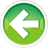# 6.1 — Compound statements (blocks)

A compound statement (also called a block, or block statement) is a group of zero or more statements that is treated by the compiler as if it were a single statement.

Blocks begin with a `{` symbol, end with a `}` symbol, with the statements to be executed being placed in between. Blocks can be used anywhere a single statement is allowed. No semicolon is needed at the end of a block.

You have already seen an example of blocks when writing functions, as the function body is a block:

Blocks inside other blocks

Although functions can’t be nested inside other functions, blocks can be nested inside other blocks:

When blocks are nested, the enclosing block is typically called the outer block and the enclosed block is called the inner block or nested block.

Using blocks to execute multiple statements conditionally

One of the most common use cases for blocks is in conjunction with `if statements`. By default, an `if statement` executes a single statement if the condition evaluates to `true`. However, we can replace this single statement with a block of statements if we want multiple statements to execute when the condition evaluates to `true`.

For example:

If the user enters the number 3, this program prints:

```Enter an integer: 3
3 is a positive integer (or zero)
Double this number is 6
```

If the user enters the number -4, this program prints:

```Enter an integer: -4
-4 is a negative integer
The positive of this number is 4
```

Block nesting levels

It is even possible to put blocks inside of blocks inside of blocks:

The nesting level (also called the nesting depth) of a function is the maximum number of nested blocks you can be inside at any point in the function (including the outer block). In the above function, there are 4 blocks, but the nesting level is 3 since in this program you can never be inside more than 3 blocks at any point.

The C++ standard says that C++ compilers should support 256 levels of nesting -- however not all do (e.g. as of the time of writing, Visual Studio supports somewhere between 100 and 110).

Despite what C++ technically supports, it’s a good idea to keep your nesting level to 3 or less. Just as overly-long functions are good candidates for refactoring (breaking into smaller functions), overly-nested functions are hard to read and are good candidates for refactoring (with the most-nested blocks becoming separate functions).

Best practice

Keep the nesting level of your functions to 3 or less. If your function has a need for more nested levels, consider refactoring your function into sub-functions.6.2 -- User-defined namespaces and the scope resolution operatorIndexO.4 -- Converting between binary and decimal

### 16 comments to 6.1 — Compound statements (blocks)

•buuface

Hello can someone help me with this bit of code. I am trying to get the console to print "Zero is not a number" if the user enters 0, and "- is a negative number" is the number is negative. Please help!

#include "stdafx.h"
#include

int main()
{
using namespace std;
cout <> nValue;

if (nValue > 0)
{ // start of nested block
if (nValue < 10)
{
cout << nValue << " is between 0 and 10" << endl;
}
cout << nValue << " is a positive number" << endl;
cout << "Double this number is " << nValue * 2 << endl;
} // end of nested block
if (nValue = 0)
{
cout << " Zero is not a fucking number!" << endl;
}
else
{
if (nValue = 0)
{
cout << " Zero is not a number!" << endl;
}
else
{
cout << nValue << " is a negative number" << endl;
cout << "double this number is " << nValue * 2 << endl;
}

}

}